# Leakage Detection Tutorial, Part 3: Statistical Interpretation

In this final part of the tutorial, we expand on some of the technical aspects of the tests that we simply took for granted in previous parts. We first look at how the decision criteria recommended by the original TVLA paper relate to the implicit statistical characteristics of the tests performed, in particular the Type I and Type II error rates. We then apply this to the task of interpreting outcomes, and offer some guidance towards responsible test design and reporting.

## Critical Values and Error Rates

Remember the threshold of 4.5 that we used in Part 1 to decide whether or not the t-statistic provided sufficient evidence for the presence of leakage? In the language of statistical hypothesis testing, this is called a critical value, and it is chosen to minimise the probability of making a certain type of wrong decision. There are two types of wrong decision an evaluator can make: she can decide that there is leakage when there isn’t (a ‘false positive’ or ‘Type I error’) or she can decide that there isn’t leakage when there is (a ‘false negative’ or ‘Type II error’). In order to understand how these error rates relate to the choice of critical value we need to rewind and look more closely at the statistical properties of the detection tests which are left implicit (and somewhat ad-hoc) within the TVLA framework.

A statistical hypothesis test confronts a ‘null hypothesis’ with observed data, to see if there is enough evidence to reject it in favour of an ‘alternative hypothesis’. In the case of leakage detection, the null hypothesis is that there is no leakage at a given point. The alternative is that there is some non-negative information leakage. A test statistic is a function of the data which is computed in such a way that it has a particular (known) distribution in the event that the null hypothesis is true. If the test statistic is outside the range of likely values for this distribution, we say that the observed evidence is ‘inconsistent with the null hypothesis’. In the case of leakage detection, the test statistic has a t-distribution in the event of zero leakage and, if it is extreme enough relative to what we expect in such a scenario, we reject the null and conclude that the trace point leaks information.

Since the t-distribution converges to the standard normal as the sample size increases, the test statistics in Part 1 are approximately normal under the null hypothesis because of the large sample sizes considered.

In :
import numpy as np
import matplotlib.pyplot as plt
import pandas as pd
import scipy.stats as sp
from IPython.display import display

In :
#Comment for DEBUG only
np.warnings.filterwarnings('ignore')

fignum = 1
plt.figure(fignum)

# Standard Normal density
x_axis = np.linspace(-6,6,120)
npdf = sp.norm.pdf(x_axis)
plt.plot(x_axis, npdf)

# Mark the TVLA thresholds on the plot:
plt.plot([-4.5,-4.5],[0,0.4],'r:')
plt.plot([4.5,4.5],[0,0.4],'r:')
plt.xlabel('Test statistic')
plt.ylabel('Density')
plt.title('Fig %.0f:\n Distribution of test statistic (standard Normal)' % fignum)
plt.show()
fignum = fignum + 1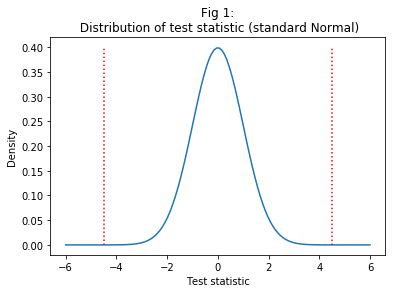The red lines on the above graph indicate the TVLA-recommended threshold of +/- 4.5 for concluding in favour of the presence of leakage. It is clear from the figure that this is quite a demanding criterion: the area underneath the right and left tails sums to a total probability of just 0.00001, which means that many still quite extreme values are considered ‘insufficient evidence’ for the presence of leakage. This has the advantage of guarding strongly against false positives: $\alpha = 0.00001$ is the probability of concluding that there is leakage when the test statistic did arise from the null distribution after all. We call it the ‘significance level’ (and $1-\alpha$ the ‘confidence level’) and it is a parameter that can be chosen by the analyst.

If the test statistic isn’t in the critical region (i.e. it isn’t larger than the threshold values relating to the chosen $\alpha$ level), then this does not prove that there isn’t leakage. From a formal statistical perspective, all it means is that “there is not enough evidence to reject, with confidence $1-\alpha$, the null hypothesis that the two sampled distributions have the same population mean”. There might be a real difference which is too small to detect with the amount of data available. At the same time, it might be that the evidence available would have been sufficient if the analyst had been more willing to risk Type I errors (that is, had she chosen a larger $\alpha$). This highlights two important considerations: 1) there is a trade-off between false positives and false negatives, and 2) the risks of both errors can be reduced by increasing the sample size.

Thus, it is not necessarily a good strategy to set the strictest possible significance criteria. True, we want to avoid errors at any cost, but depending on the particular priorities of the analysis, we need to be just as attentive to the Type II error rate $\beta$ as to the Type I error rate $\alpha$. That is, we want to be able to make conclusions with high ‘power’ (the power of a test is the probability of a true positive, defined as $1-\beta$) as well as high confidence.

Understanding both types of errors and how they interact is important for designing tests which are fit for purpose. It is also, as we will discuss first, important for interpreting test outcomes, especially in the event that the null hypothesis (of ‘no leakage’, in our case) is not rejected.

## What if a Test Fails to Find Leakage?

With the above in mind, then, suppose you perform a t-test for the leakage of bit $b$ at point $t^*$ using $N$ traces, and your test statistic is below the threshold. What does this tell you?

Well, formally, as noted above, it tells you that “there is not enough evidence to reject, with confidence $1-\alpha$, the null hypothesis that the distributions for $b=0$ and $b=1$ have the same mean”.

In practice: it might mean that point $t^*$ doesn’t leak bit $b$… but it might just as well be that the true difference of means $\zeta = \mu_{b=0} – \mu_{b=1}$ requires more data to detect than you currently have available, at least without increasing the risk of drawing an incorrect conclusion in the event that the difference is actually zero (a ‘false positive’, or ‘Type I error’, denoted $\alpha$).

The smaller the true difference (relative to the amount the measurements inevitably vary), the more observations you need in order to be confident that any observed difference is not just the result of imprecise estimation.

Don’t forget, the observed difference is highly unlikely to be exactly zero, even if the true difference is, because of estimation error. The criteria to decide whether the difference is “non-zero enough” can feel cumbersome and opaque, but they are necessary tools if we want to draw well-reasoned conclusions which remain comparable from one evaluation to another.

## Deriving the Minimum Effect Size

One thing you can do after a ‘failed’ leakage detection is explore how big the effect would have to be for your dataset to have revealed it with reasonable probability. Let’s call this quantity $\zeta_{min}$.

If $\zeta_{min}$ is small enough to be ‘uninteresting’ — that is, if you judge a leak of that magnitude to be not worth worrying about — then you might be reassured that you have done enough to certify security. Although the hypothesis test does not enable you to ‘accept’ the null of no leakage, you can use the value of $\zeta_{min}$ to reason for the adequacy of your analysis.

Calculating $\zeta_{min}$ generally requires making some simplifying assumptions about the distributions, fixing your desired error probabilities, and deriving the appropriate formulae using the methods of ‘statistical power analysis’. This is not to be confused with ‘power analysis’ in the side-channel sense; statistical power means the probability of detecting a true effect, which is equivalent to $1-\beta$ where $\beta$ is the rate of false negatives (a.k.a.\ Type II errors).

Power analysis involves the relationship between the power, the confidence level (which controls the rate of false positives), the effect size, and the sample size. Fixing any three of these determines the fourth.

For now, we are interested in finding the effect size when the confidence level and the sample size are as per the ‘failed’ t-test. First we have to decide how powerful we need our test to be — or we could compute different minimum effect sizes for a range of powers.

The formula for the minimum effect size, under the assumption that the two subsamples induced by bit $b$ have the same size $N/2$ and are normally distributed with variances $\sigma_{b = 0}^2$ and $\sigma_{b = 1}^2$, is as follows:

$$\zeta_{min} =(z_{\beta} + z_{\alpha / 2}) \cdot \sqrt{\frac{2}{N} \cdot (\sigma_{b=0}^2 + \sigma_{b=1}^2)}$$.

Unfortunately the variances are usually unknown and have to be substituted with estimates $\hat{\sigma}_{b=0}^2$ and $\hat{\sigma}_{b=1}^2$. Ideally these will be obtained independently of the test sample (for example, from previous work), but this is not always possible.

Suppose that our test controlled the rate of false positives at $\alpha = 0.05$ (a popular choice in other statistical applications; we will return to the stricter TVLA criterion later), that it had a sample size of $N=10000$, and that we want to balance the rate of false positives and false negatives, implying a probability of 0.95 of detecting a true effect. Suppose further that, from previous studies, we know the variance to be around 3, and to be the same for both subsamples.

In :
N = 10000
alpha = 0.05
power = 0.95
var_0 = 3
var_1 = 3

zeta_min = np.sqrt((2/N)*np.square((sp.norm.ppf(1-alpha/2) + sp.norm.ppf(power)))*(var_0 + var_1))

print('Minimum effect size: %.4f.' % zeta_min)

Minimum effect size: 0.1249.


Is this effect ‘large enough to be interesting’? Making such judgments requires expertise and experience. If we consider that it is, we will probably want to repeat the experiment with a larger sample. We can work out how large the new sample needs to be by rearranging the formula for efffect size and plugging in a chosen value $\zeta_{min} = \zeta_{ch}$
which represents our best lower bound on ‘interesting’ (e.g. exploitable by an attacker) effects.

$$N = 2\cdot\frac{(z_{\alpha/2}+z_\beta)^2\cdot({\sigma_{b=0}}^2 + {\sigma_{b=1}}^2)}{\zeta_{ch}^2}$$.

Suppose we want to be able to find effects of a tenth of the size, $\zeta_{ch} = \zeta_{min}/10$.

In :
zeta_ch = zeta_min/10
N_new = 2*np.square((sp.norm.ppf(1-alpha/2) + sp.norm.ppf(power))) * (var_0 + var_1)/np.square(zeta_ch)

print('Sample size needed to detect an effect size of %.4f: %.0f.' % (zeta_ch,N_new))

Sample size needed to detect an effect size of 0.0125: 1000000.


In other words, detecting an effect a tenth of the size requires 100 times as many traces.

Remember that, since hypothesis tests rely on estimates, which themselves are realisations of random variables, this does not guarantee that a test of the size N_new will find an effect of size zeta_ch finding an effect of that size. Rather, it controls the probability of finding such an effect (if it exists) at power = 0.9.

## Standardised Effect Sizes

There is no getting away from the fact that interpreting effect sizes is difficult. However, standardised effect sizes may help when it comes to comparing between studies.

Cohen’s $d$ is defined as the raw effect size $\zeta$ divided by the pooled standard deviation:

$$d = \frac{\zeta}{\sqrt{\frac{1}{2}(\sigma_{b=0}^2 + \sigma_{b=1}^2)}}$$.

(The denominator in the above is the pooled population standard deviation under the assumption of equal samples. The expression is more complex if the standard deviations need to be estimated, and/or if the samples are different sizes).

The formula for the sample size can be re-written in terms of the standardised effect size as follows:

$$N = 4\cdot\frac{(z_{\alpha/2}+z_\beta)^2}{\zeta_{ch}^2}$$.

Returning to our running example, we can compute the standardised effects
as follows:

In :
pooledSD = np.sqrt( (var_0 + var_1) / 2)
d_min = zeta_min/pooledSD

print('Minimum standardised effect detectable in existing sample: %.4f.' % d_min)

pooledSD_new = np.sqrt((var_0 + var_1) / 2)
d_new = zeta_ch/pooledSD_new

print('Minimum standardised effect detectable in planned new experiment: %.4f.' % d_new)

Minimum standardised effect detectable in existing sample: 0.0721.
Minimum standardised effect detectable in planned new experiment: 0.0072.


Cohen [Coh1988] proposed that effects of 0.2 or less should be considered ‘small’, effects around 0.5 are ‘medium’, and effects of 0.8 or more are ‘large’. Sawilowsky [Saw2009] expanded the list to incorporate ‘very small’ effects of 0.01 or less, and ‘very large’ and ‘huge’ effects of over 1.2 or 2.0 respectively.

However, care should be taken applying these generalised recommendations to the side-channel setting, as the magnitude and seriousness of typical effects depends on the application. For example, unless countermeasures limit the number of uses of a particular key, large samples of leakage traces can often be acquired very cheaply relative to studies in other fields such as medicine, psychology and econometrics, where each observation might rely on an experimental subject, a survey respondent or an historic record which cannot be supplemented after the fact. This, combined with the high security stakes of side-channel analysis, make ‘very small’ effects of more interest than they might be elsewhere. (See, for example, [DEM2018] in which detection tests are performed using as many as 500 million traces).

### Graphing the relationship between sample size, effect size and power

This code computes and plots power for increasing sample sizes:

In :
#'Very small' through to 'huge' effects as per Cohen and Sawilowsky:
stdeff = np.array([0.01, 0.2, 0.5, 0.8, 1.2, 2])
#Compute the corresponding 'raw' effects in the context of our running example:
efflist = stdeff*np.sqrt(0.5*var_0 + 0.5*var_1)
#Legend labels:
labellist = ['Very small', 'Small', 'Medium', 'Large', 'Very large', 'Huge']
for i in range(0, len(labellist), 1):
labellist[i] +=' (%.2f)' % efflist[i]

#Significance level:
alpha = 0.05
#Selected sample sizes at which to evaluate:
Nlist = np.concatenate((np.linspace(1,30,30), np.linspace(40,10000,997), np.linspace(10100,1000000,9900)))
#Initialise array in which to store power computations:
power = np.zeros([len(stdeff), len(Nlist)])

#Compute power using the fomula:
for n in range(0, len(Nlist), 1):
for se in range(0, len(stdeff), 1):
power[se,n] = sp.norm.cdf( np.sqrt(Nlist[n]*(stdeff[se]**2) / 4) - sp.norm.ppf(1 - alpha/2))

plt.figure(fignum)
plt.clf()
plt.plot(np.log10(Nlist), power.T)
plt.xlim(0,  np.log10(Nlist[-1]))
plt.legend(labellist, loc='upper left', bbox_to_anchor=(1, 1))
plt.xlabel('log10(Sample size)')
plt.ylabel('Power')
title_str = ('Fig %.0f: Power as sample size increases\n' % fignum) + (r'$\alpha$ = %.2f' % alpha)
plt.title(title_str)
plt.show()
fignum = fignum + 1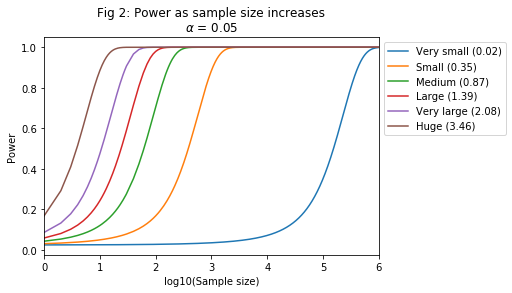This code computes and plots effect sizes for increasing sample sizes:

In :
#Consider a range of possible desired powers:
powerlist = np.array([0.75, 0.8, 0.9, 0.95, 0.99])
#Selected sample sizes at which to evaluate:
Nlist = np.concatenate((np.linspace(1,30,30), np.linspace(40,10000,997), np.linspace(10100,1000000,9900)))
#Significance level:
alpha = 0.05
#Initialise array to store the computed effect sized:
eff = np.zeros([len(powerlist), len(Nlist)])
#Compute the effect sizes using the formula:
for n in range(0, len(Nlist), 1):
for p in range(0, len(powerlist), 1):
eff[p,n] = np.sqrt( (2/Nlist[n]) * ( (sp.norm.ppf(alpha/2) + sp.norm.ppf(1 - powerlist[p]))**2) * (var_0 + var_1) )

#Find the raw effect sizes that correspond to Cohen and Sawilowsky's standardised
#effects:
stdeff = np.array([0.01, 0.2, 0.5, 0.8, 1.2, 2])
threshold = stdeff * np.sqrt(0.5*var_0 + 0.5*var_1)

#Legend labels
leglist = []

for p in range(0, len(powerlist), 1):
leglist.append('Power = %.2f' % powerlist[p])

for t in range(0, len(threshold), 1):
leglist.append('d = %.2f' % stdeff[t])

plt.figure(fignum)
plt.clf()
plt.plot(np.log10(Nlist), eff.T)
for i in range(0, len(threshold), 1):
plt.plot([np.log10(Nlist), np.log10(Nlist[-1])], [threshold[i], threshold[i]], ':')

plt.legend(leglist, loc='upper right', bbox_to_anchor=(1.5, 1))
plt.xlabel('log10(Sample size)')
plt.ylabel('Raw effect')
title_str = ('Fig %.0f: Smallest detectable effects\n' % fignum) + (r'$\alpha$ = %.2f' % alpha)
plt.title(title_str)
fignum = fignum + 1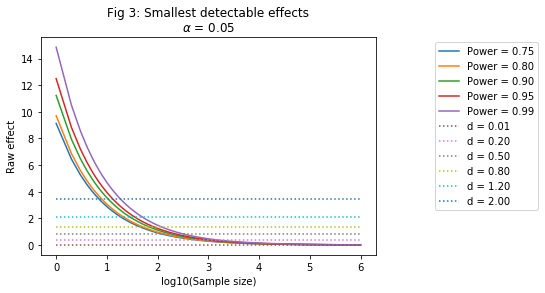## Planning a Test with the Desired Properties

An evaluator need only decide on the effect size she is interested in and the power that the test is required to have and from that can fix the sample size in advance, so that the experiment will be fit for purpose. Using our running example scenario, we construct a table showing sample size for different experimental designs:

In :
#Sample size needed for effects of interest:
powerlist = np.array([0.75, 0.8, 0.9, 0.95, 0.99])
labellist = ['Very small', 'Small', 'Medium', 'Large', 'Very large', 'Huge']
#Compute the corresponding 'raw' effects in the context of our running example:
efflist = stdeff*(np.sqrt(0.5*var_0 + 0.5*var_1))
N = np.zeros([len(stdeff), len(powerlist)])
for i in range(0, len(stdeff), 1):
for p in range(0, len(powerlist), 1):
N[i,p] = (4/stdeff[i]**2) * ((sp.norm.ppf(1-alpha/2) + sp.norm.ppf(powerlist[p]))**2)

#Display table:
tabnum = 1
for i in range(0,len(powerlist),1):

print('Tab %.0f: Sample sizes required to detect effects of size d at significance levels of %.2f.' % (tabnum, alpha))
pd.options.display.float_format = '{:.4g}'.format
display(df)
tabnum = tabnum + 1

Tab 1: Sample sizes required to detect effects of size d at significance levels of 0.05.

StdEffect RawEffect Power = 0.75 Power = 0.80 Power = 0.90 Power = 0.95 Power = 0.99
Very small 0.01 0.01732 2.776e+05 3.14e+05 4.203e+05 5.198e+05 7.349e+05
Small 0.2 0.3464 694 784.9 1051 1299 1837
Medium 0.5 0.866 111 125.6 168.1 207.9 294
Large 0.8 1.386 43.38 49.06 65.67 81.22 114.8
Very large 1.2 2.078 19.28 21.8 29.19 36.1 51.03
Huge 2 3.464 6.94 7.849 10.51 12.99 18.37

### Changing the significance level

Until now we have treated the significance level as fixed, but in reality it is another parameter that can be varied in order to tune the experiment to suit the goals of the evaluation. Suppose for example that we want to return to the very low rate of false positives (1 in every 10,000) implied by the threshold criteria proposed for TVLA. Let’s look at the impact of this stricter requirement on the sample sizes required for the tests above:

In :
alpha_TVLA = 0.00001
N_TVLA = np.zeros([len(stdeff), len(powerlist)])
for i in range(0, len(stdeff), 1):
for p in range(0, len(powerlist), 1):
N_TVLA[i,p] = (4/stdeff[i]**2) * ((sp.norm.ppf(1-alpha_TVLA/2) + sp.norm.ppf(powerlist[p]))**2)

#Display table:
for i in range(0,len(powerlist),1):

print('Tab %.0f: Sample sizes required to detect effects of size d at significance levels of %.5f.' % (tabnum, alpha_TVLA))

pd.options.display.float_format = '{:.4g}'.format

display(df)
tabnum = tabnum + 1

Tab 2: Sample sizes required to detect effects of size d at significance levels of 0.00001.

StdEffect RawEffect Power = 0.75 Power = 0.80 Power = 0.90 Power = 0.95 Power = 0.99
Very small 0.01 0.01732 1.037e+06 1.106e+06 1.299e+06 1.47e+06 1.819e+06
Small 0.2 0.3464 2593 2765 3248 3675 4548
Medium 0.5 0.866 414.8 442.5 519.6 588 727.6
Large 0.8 1.386 162 172.8 203 229.7 284.2
Very large 1.2 2.078 72.01 76.82 90.21 102.1 126.3
Huge 2 3.464 25.93 27.65 32.48 36.75 45.48

Equivalently, the tests vecome considerably less powerful for a given sample size. Compare the following figures with those aboce for $\alpha = 0.05$:

In :
#Initialise array in which to store power computations:
power = np.zeros([len(stdeff), len(Nlist)])
#Compute power using the formula:
for n in range(0, len(Nlist), 1):
for se in range(0, len(stdeff), 1):
power[se, n] = sp.norm.cdf( np.sqrt((Nlist[n]*stdeff[se]**2)/4 )  - sp.norm.ppf(1 - alpha_TVLA/2))

plt.figure(fignum)
plt.clf()
plt.plot(np.log10(Nlist), power.T)
plt.xlim(0,  np.log10(Nlist[-1]))
plt.legend(labellist, loc='upper right', bbox_to_anchor=(1.35,1))
plt.xlabel('log10(Sample size)')
plt.ylabel('Power')
title_std = ('Fig %.0f: Power as sample size increases\n' % fignum) + (r'$\alpha$ = %.5f' % alpha_TVLA)
plt.title(title_std)
fignum = fignum + 1

#Compute and plot effect sizes for increasing sample sizes:
#Initialise array to store the computed effect sizes:
eff = np.zeros([len(powerlist), len(Nlist)])
#Compute the effect sizes using the formula
for n in range(0, len(Nlist), 1):
for p in range(0, len(powerlist), 1):
eff[p,n] = np.sqrt( (2/Nlist[n]) * ( ((sp.norm.ppf(1-alpha_TVLA/2) +
sp.norm.ppf(powerlist[p]))**2) * (var_0 + var_1) ))

plt.figure(fignum)
plt.clf()
plt.plot(np.log10(Nlist),eff.T)
plt.xlim(0, np.log10(Nlist[-1]))
for i in range(0, len(threshold), 1):
plt.plot([np.log10(Nlist), np.log10(Nlist[-1])], [threshold[i], threshold[i]],':')

plt.legend(leglist, loc='upper right', bbox_to_anchor=(1.35,1))
plt.xlabel('log10(Sample size)')
plt.ylabel('Raw effect')
title_std = ('Fig %.0f: Smallest detectable effects\n' % fignum) + (r'$\alpha$ = %.5f' % alpha_TVLA)
plt.title(title_std)
fignum = fignum + 1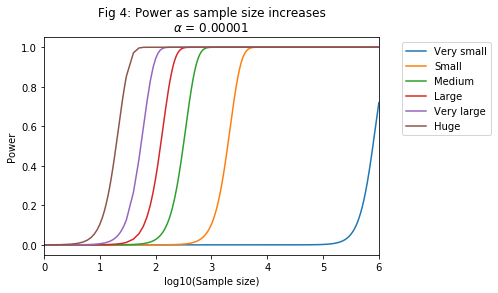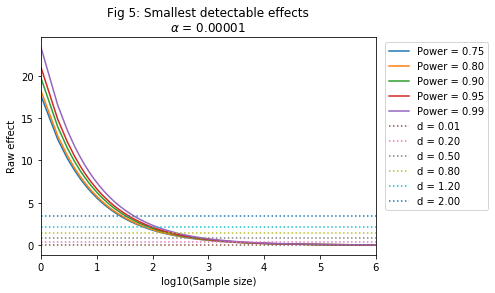The tendency in the side-channel literature has been to only consider false positives and to set a very small $\alpha$ accordingly. But the smaller the $\alpha$, the more evidence needed to conclude that there is a vulnerability of a given size. A high burden of proof may not always reflect the actual goals of the evaluation: it might sometimes be just as bad (or worse) to miss a leak that is there as it is to find one that isn’t. And side-channel analysis is one setting where small but real differences really matter: with the right attack they can lead to the same amount of secret information leakage as a large difference. Designing an evaluation ideally involves clarifying one’s priorities beforehand and choosing parameters with these various trade-offs in mind.

## What Does This Look Like In Practice?

Having established some theoretical characteristics of the statistical procedures which form the basis of popular leakage detection tests, we now wish to see how these play out in practice. In the following, we will imagine a range of scenarios with differing effect sizes (including one with no effect at all). We will first use the tools of statistical power analysis to determine the requirements and expected performance of the tests. We will then perform the test against simulated (univariate) leakages. Because statistical power analysis offers guarantees about error rates (i.e. average tendencies) a single experiment tells us very little; we must perform numerous repetitions in order to estimate the proportions of false positives and negatives and to compare them with the projections obtained a priori. (N.B. The necessity to perform repeat experiments makes the following code more computationally heavy than the preceding snippets).

### Table of sample sizes needed to detect standardised effects of interest

In :
#Selected values of Cohen's d (including a 'zero effect' for comparison;
#we restrict to small and very small values as these are of more relevance
#to the context of side-channel leakage):
dlist = np.array([0.0, 0.01, 0.2, 0.5, 0.8])
#Different significance levels for the test:
alphalist = np.array([0.00001, 0.01, 0.05])
#Desired power
power = 0.95

#Compute the sample size for the specified values of d at the specified
#significance levels with the desired power.
N = np.empty([len(dlist), len(alphalist)])
N[:] = np.nan

for d in range(1, len(dlist), 1):
for a in range(0, len(alphalist), 1):
N[d,a] = np.ceil(( 4 * ( sp.norm.ppf(1-alphalist[a]/2) + sp.norm.ppf(power) )**2 ) / (dlist[d]**2))

for aa in range(1, len(alphalist), 1):

labellist.clear()
for dd in range(0, len(dlist), 1):
labellist.append('d = %.2f' % dlist[dd])

print('Tab %.0f: Sample sizes required to detect effects of size d at significance levels of alpha.' % tabnum)
pd.options.display.float_format = '{:.0f}'.format
display(df)
tabnum = tabnum + 1

Tab 3: Sample sizes required to detect effects of size d at significance levels of alpha.

TVLA alpha = 0.01 alpha = 0.05
d = 0.00 nan nan nan
d = 0.01 1469927 712567 519789
d = 0.20 3675 1782 1300
d = 0.50 588 286 208
d = 0.80 230 112 82

The table above shows the Python output for a sample size computation (to achieve a power of 0.95) for three different values of $\alpha$ and the five different values of $d$ that we are interested in.

The first column shows the sample size required under the de facto significance criteria of $\alpha = 0.00001$ implied by the TVLA recommendations. For the ‘very small’ standardised effect this is in the order of millions — a familiar figure from practical experience if the side-channel literature is anything to go by (see, e.g. [DEM2018]). The second and third columns correspond to popular choices of $\alpha$ in statistical applications more generally.

### A single experiment

The following code performs a single experiment with a particular $d$ and $\alpha$ in mind. We suggest experimentally modifying these parameters to produce tests with different outcomes. Think about what outcomes you expect based on the changes you are making. Try using the statistical power analysis formulae to shape your expections and design tests that you think will or won’t be able to detect the leakages present.

First we configure the leakage and test scenario and simulate the trace
measurements:

In :
#Significance level:
alpha = 0.05
#Cohen's d:
d = 0.2
#Sample size:
samplesize = 2000

#Random generate a set of intermediate values:
intvals = np.random.randint(0, 256, (samplesize, 1), dtype=np.uint8)
#Leakage with a standardised effect of d can be simulated by mulitplying the
#target bit (1 -> MSB, 8 -> LSB) by d and adding standard normal noise:
bin_intvals =  np.reshape(np.unpackbits(intvals, axis=1), (samplesize, 8))
simtrace = np.reshape(d*bin_intvals[:,0],(samplesize,1)) + np.random.randn(len(intvals),1)

#Partition the simulated trace set:
set0 = simtrace[bin_intvals[:,0]==0]
set1 = simtrace[bin_intvals[:,0]==1]


Next we plot the distributions of the two subsets of traces. Running this a few times with the same parameters will give a sense of the natural variability:

In :
plt.figure(fignum)
plt.clf()
ind = np.linspace(np.min(set0), np.max(set0),100)
kde0 = sp.gaussian_kde(set0.T)
kde1 = sp.gaussian_kde(set1.T)
plt.plot(ind, kde0.evaluate(ind))
plt.plot(ind, kde1.evaluate(ind))
plt.title('Fig %.0f: Kernel density of partitioned trace\n (True effect size: %.2f)' % (fignum,d))
plt.xlabel('Trace value')
plt.ylabel('Density')
plt.legend(['Target bit = 0', 'Target bit = 1'], loc='upper right')
fignum = fignum + 1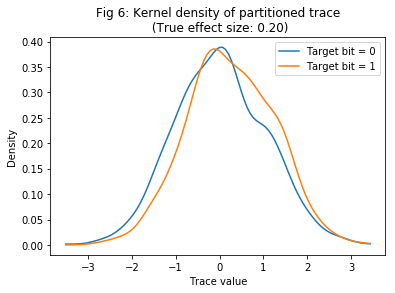Next, we compute the t-statistic and compare it against the critical value of the appropriate t-distribution. This entails calculating the ‘degrees of freedom’ (DoF) from the sample sizes and sample variances, via the Welch-Satterthwaite equation. A detailed explanation of DoFs is outside the scope of this tutorial; think of it as a quantity which characterises the shape of the null distribution. As the sample size increases, the t-distribution tends towards the standard normal and the degrees of freedom becomes irrelevant. Since many leakage detection scenarios involve small effects and correspondingly large samples, it is usually safe to skip straight to the approximation (the TVLA critical value of 4.5, for example, assumes that convergence has already happened). However, for the purposes of allowing experiments to be made with smaller samples we provide the exact t-test version.

In :
#Count the number in each subset:
N0 = len(set0)
N1 = len(set1)

#Compute the sample variance of each subset:
s0_2 = np.var(set0)
s1_2 = np.var(set1)

#Compute the t-statistic:
tStatistic = (np.mean(set1) - np.mean(set0)) / np.sqrt(s0_2/N0 + s1_2/N1)
#Compute the degrees of freedom for the Welch's t-test:
dof = np.round(( s0_2/N0 + s1_2/N1 )**2 / ( (s0_2**2)/(N0*N0*(N0-1)) + (s1_2**2)/(N1*N1*(N1-1)) ))

#Find the threshold at which to reject the null hypothesis of 'no effect' in
#favour of the alternative hypothesis that there is an effect:
thr = sp.t.ppf(1-alpha/2,dof)

#Display results:
print('Standardised difference: d = %.2f; sample size: N = %.2f; significance criterion: alpha = %f.'
% (d, samplesize, alpha))
print('t-statistic: %.2f; DoF: %.0f; critical value: %.2f.' % (tStatistic, dof, thr))

if np.abs(tStatistic) > thr:
print('Null is rejected at the %f level.' % alpha)
else:
print('Null is not rejected at the %f level.' % alpha)

print('Try adjusting the parameters (alpha, d, N) to see how this changes the outcome.')

Standardised difference: d = 0.20; sample size: N = 2000.00; significance criterion: alpha = 0.050000.
t-statistic: 5.11; DoF: 1995; critical value: 1.96.
Null is rejected at the 0.050000 level.
Try adjusting the parameters (alpha, d, N) to see how this changes the outcome.


### Repeat experiments to look at long-run behaviour

With the parameters set by careful design, we hope that the above experiment will give outcomes which reflect the known (because simulated) ground truth “most of the time”. But errors are possible, and there is no way of observing the error rates from a single actual test. We would have to repeat the whole experiment a large number of times in order to obtain these — which is not an option in a real and unknown testing environment (not to mention that we have no ground truth knowledge against which to assess the correctness of our test outcomes). It is precisely because we can’t observe error rates in practice that we need reliable theory to help us control them.

However, in this artificial simulated environment we can repeat the experiments, if we wish, in order to verify that the error rates are as we expect them to be, and to give us confidence in the theory.

To keep computational costs within reasonable bounds, we will consider significance levels $\alpha = 0.05$. We use the sample sizes obtained in the above analysis (see Table 3) to derive a meaningful range of increasing sample size values at which to compute the theoretical power and, by way of comparison, experimentally obtain the the proportion of successful detections.

Because of the number of experiments performed this section of the script takes considerably longer to run than the preceding cells.

In :
#We wish to compare between different significance level:
alpha = 0.05
#We compare across a range of values of Cohen's d, as before:
dlist = np.array([0.0, 0.01, 0.2, 0.5, 0.8])
#Construct meaningul sample size ranges on which to perform the experiments:
#We have already (see Table 3) derived a minimum sample sizes for detection
#using the techniques of statistical power analysis. (In the case of zero
# effects, we take a large upper bound at which to demonstrate non-detection).
N = np.array([1000000,519789,1300,208,82])
#Guided by the above, set the maximum sample sizes at which to rund the
#experiments:
maxN = np.array([10000000,1000000,4000,500,300])
#Construct experiment-specific sample size lists to have 15 equally
#spaced evaluation points on a log scale. We also want to include the following
#* 4 as minimum sample size;
#* the overall maximum;
#* the particular sample sizes derived in Table 3.
nlist = [None]*(len(dlist))
for d in range(0, len(dlist), 1):
inc = np.log10(maxN[d])/15
nlist[d] = (np.unique(np.hstack([4, np.ceil(10**np.arange(inc,np.ceil(np.log10(maxN[d])), inc)),
maxN[d],np.max(maxN), N[d]])))

#Number of repetitions to perform:
reps = 200

#Initialise cell arrays in which to store the generated outcomes:
#Significan effect found or not (indicator):
sigeff = [None]*len(nlist) # np.empty([len(dlist), len(alphalist)])
#Observed effect size:
esteffect = [None]*len(nlist)  #np.empty([len(dlist), len(alphalist)])
#t-statistic:
tStatistic = [None]*len(nlist) # np.empty([len(dlist), len(alphalist)])
#Degrees of freedom for Welch's t-test:
dof = [None]*len(nlist)  #np.empty([len(dlist), len(alphalist)])
#Power of the test:
power_t = [None]*len(nlist)  #np.empty([len(dlist), len(alphalist)])
#Initialise the outcome arrays to zeros:
for i in range(len(nlist)):
sigeff[i] = np.zeros((reps,len(nlist[i])))
esteffect[i] = np.zeros((reps,len(nlist[i])))
tStatistic[i] = np.zeros((reps,len(nlist[i])))
dof[i] = np.zeros((reps,len(nlist[i])))
power_t[i] = np.zeros(len(nlist[i]))

#Run repeat experiments to get (observed and theoretical) power as sample size
#increases:
for r in range(0, reps, 1):
if np.mod(r+1,10)==0:
print('Repetition %.0f of %.0f' % (r+1,reps))
#Loop over different effect sizes:
for d in range(0, len(dlist), 1):
#Simulate a point in the traces with the speficied standardised
#effect size:
intvals = np.random.randint(0, 256, size=(np.max(maxN),1), dtype=np.uint8)
bin_intvals =  np.reshape(np.unpackbits(intvals, axis=1), (np.max(maxN), 8))
simtrace = np.reshape(dlist[d]*bin_intvals[:,0],(np.max(maxN),1)) + np.random.randn(len(intvals),1)
for n in range(0, len(nlist[d]), 1):
#Take the first nlist[d](n) observations:
tr = simtrace[0:int(nlist[d][n]):1]
iv = bin_intvals[0:int(nlist[d][n]):1]
#Partition the trace measurements according to the intermediate
#bit
set0 = tr[iv[:,0]==0,0]
set1 = tr[iv[:,0]==1,0]
#Subset sizes:
N0 = len(set0)
N1 = len(set1)
#Subset sample variances:
s0_2 = np.var(set0)
s1_2 = np.var(set1)
#Pooled standard deviations:
pooledsd = np.sqrt((s0_2*(N0-1) + s1_2*(N1-1))/(N0+N1-2))
#t-statistic:
tStatistic[d][r][n] = (np.mean(set1) - np.mean(set0)) / np.sqrt(s0_2/N0 + s1_2/N1)
#Degrees of freedom:
dof[d][r][n] = np.round((( s0_2/N0 + s1_2/N1 )**2)/( (s0_2**2)/(N0*N0*(N0-1)) +
(s1_2**2)/(N1*N1*(N1-1))))
#Find the critical value of the appropriate t-distribution:
thr = sp.t.ppf(1-alpha/2,dof[d][r][n])
#Is the t-statistic large enough to reject the null hypothesis?
sigeff[d][r][n] = np.abs(tStatistic[d][r][n]) > thr
#Compute the estimated standardised effect size:
esteffect[d][r][n] = (np.mean(set1) - np.mean(set0)) / pooledsd

#Derive the theoretical power by statistical power analysis
#(for comparison):
if r==0:
power_t[d][n] = sp.norm.cdf((np.sqrt(N0+N1)*dlist[d])/2  + sp.norm.ppf(alpha/2) )

Repetition 10 of 200
Repetition 20 of 200
Repetition 30 of 200
Repetition 40 of 200
Repetition 50 of 200
Repetition 60 of 200
Repetition 70 of 200
Repetition 80 of 200
Repetition 90 of 200
Repetition 100 of 200
Repetition 110 of 200
Repetition 120 of 200
Repetition 130 of 200
Repetition 140 of 200
Repetition 150 of 200
Repetition 160 of 200
Repetition 170 of 200
Repetition 180 of 200
Repetition 190 of 200
Repetition 200 of 200


### Plot the outcomes of the above analysis

This code produces plots of the theoretical power and estimated proportion of detections as the sample size increases (on a log scale). Separate figures are produced for each of the tested significance criteria.

In :
leglist = []

plt.figure(fignum)
plt.clf()
cols = []
for d in range(0, len(dlist),1):
h = plt.plot(np.log10(nlist[d]), np.mean(sigeff[d],axis=0))
cols.append(h.get_color())
for d in range(0, len(dlist),1):
plt.plot(np.log10(N[d]), np.mean(sigeff[d][:,np.argwhere(nlist[d]==np.ceil(N[d]))]), 'ko')
plt.plot(np.log10(nlist[d]), power_t[d],':', color=cols[d])
#Plot the power aimed for in the theoretical analysis of Table 3:
h = plt.plot([np.log10(4), np.log10(np.max(maxN))], [0.95, 0.95],':k')
plt.xlabel('Sample size')
plt.ylabel('Proportion of nulls rejected')
plt.xticks(np.linspace(1,6,6),10**np.linspace(1,6,6))
newaxis = np.asarray(plt.axis())
newaxis = np.max([newaxis, np.log10(5)])
newaxis = np.log10(np.max(maxN))
plt.axis(newaxis)
title_str = 'Fig %.0f: Detection rate when ' % fignum + r'$\alpha$ = %.2f' % alpha + \
'\n Theoretic (dashed line) and observed (solid line)'
plt.title(title_str)
if a == 0:
for d in range(0, len(dlist), 1):
leglist.append('d = %.2f' % dlist[d])
leglist.append('Power = 0.95')
plt.legend(leglist, bbox_to_anchor=(1,1))
plt.show()
fignum = fignum + 1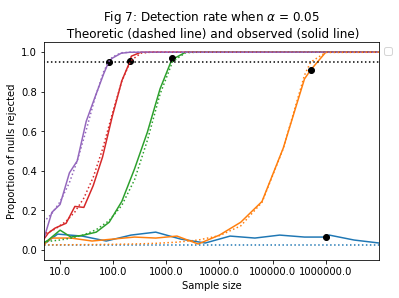The above graph shows that the observed detection rates track the
theoretic computations well, and that the 95% detection rates are reached
around about the sample sizes that we projected they would be.

The observed and theoretic detection rates are, as we would expect,
greater for the larger significance level. However, so too is the rate of
false positives (naturally, as this is precisely what the significance
level seeks to control).

## Multiple Comparisons and Overall Error Rates

The discussion and analysis so far in this part of the tutorial has given
little consideration to the fact that real trace acquisitions comprise
large numbers (often thousands) of trace points, and that detection tests
are typically applied to each one of these separately in order to see if
there is leakage anywhere and to locate it correctly. It turns out that
this is not something we can safely ignore.

Let’s first make the simplifying (almost certainly unrealistic)
assumption that the trace points, and therefore the tests, are
independent. Then, if the per-test probability of a Type I error (i.e. a
false detection) is $\alpha_{per-test}$, the overall probability can be
derived as $\alpha_{overall} = 1 – (1 – \alpha_{per-test})^L$, where $L$
is the length of the traces. Let’s see what this looks like for the
values of $\alpha$ considered earlier in the script, and some realistic
trace lengths:

In :
#Per-test significance criteria:
alpha_pertest = np.array([0.05,0.01,0.00001]).T
#Range lengths:
L = np.array([1, 10, 50, 100, 1000, 5000, 100000])
#Compute and display overall false detection rates (under the assumption of
#independence):
print('Tab %.0f: Overall false detection rates under the assumption of independence.' % tabnum)

labellist = []
for a in range(0, len(alpha_pertest), 1):
labellist.append('PerTestRate = %.4f' % alpha_pertest[a])

for l in range(0,len(L),1):

alpha_pertest_tile = np.tile( np.reshape(alpha_pertest, (len(alpha_pertest),1)) , (1,len(L)) )
df = pd.DataFrame(data=1-np.power((1-alpha_pertest_tile),np.tile(L,(len(alpha_pertest),1))),

pd.options.display.float_format = '{:.4g}'.format
display(df)
tabnum = tabnum + 1

Tab 4: Overall false detection rates under the assumption of independence.

Rate_L1 Rate_L10 Rate_L50 Rate_L100 Rate_L1000 Rate_L5000 Rate_L100000
PerTestRate = 0.0500 0.05 0.4013 0.9231 0.9941 1 1 1
PerTestRate = 0.0100 0.01 0.09562 0.395 0.634 1 1 1
PerTestRate = 0.0000 1e-05 0.0001 0.0004999 0.0009995 0.00995 0.04877 0.6321

It is immediately obvious from the above that setting $\alpha = 0.05$ (as
is a popular choice in many statistical applications) is disastrous for
overall Type I error rates. The very small significance criteria implied
by the TVLA recommendations fares much better: even for a trace of length
5000 the overall error is still controlled below 0.05. However, as trace
lengths continue to increase we eventually need to be concerned.

A simple ‘fix’ for the multiple comparisons problem is to adjust the
per-test criterion in such a way that the overall error rate is
controlled as desired. For example, if the tests are truly independent
then setting $\alpha_{per-test} = \frac{\alpha_{overall}}{L}$ (the
‘Bonferroni correction’) will achieve this. It might be noted that the
TVLA recommendation in effect amounts to an ad-hoc correction that, for
trace lengths in the single-digit thousands, implicitly controls the
overall error rate at or around 0.05. The TVLA requirement to repeat the
entire detection procedure on a second independent acquisition, and
retain only those trace points which were identified in both instances,
is a further strong guard against false detections, effectively squaring
the per-test rate so that the overall rate is $\alpha_{overall} = 1 – (1 • \alpha_{per-test}^2)^L$:
In :
print('Tab %.0f: Overall false detection rates when no adjustment is made but the experiment is repeated.' % tabnum)
alpha_pertest_tile = np.tile( np.reshape(alpha_pertest, (len(alpha_pertest),1)) , (1,len(L)) )
df = pd.DataFrame(data=1-np.power((1-alpha_pertest_tile**2),np.tile(L,(len(alpha_pertest),1))),

pd.options.display.float_format = '{:.4g}'.format
display(df)

Tab 5: Overall false detection rates when no adjustment is made but the experiment is repeated.

Rate_L1 Rate_L10 Rate_L50 Rate_L100 Rate_L1000 Rate_L5000 Rate_L100000
PerTestRate = 0.0500 0.0025 0.02472 0.1176 0.2214 0.9182 1 1
PerTestRate = 0.0100 0.0001 0.0009996 0.004988 0.009951 0.09517 0.3935 1
PerTestRate = 0.0000 1e-10 1e-09 5e-09 1e-08 1e-07 5e-07 1e-05

It could perhaps be argued that, within the range of test scenarios
typical to side-channel evaluation, the TVLA recommendations are as good
a solution to inflated Type I error rates as are standard multiplicity
corrections. Of course, as should be clear from the preceding parts of
this script, both are bound to induce a corresponding increase in false
negatives (that is, failures to detect leakages which are actually
present; although it should be noted that the probability of detecting
at least one leak grows as the number of true leaks present in the
trace increases so, depending on the particular requirements of the
evaluation, testing more points has the potential to help as well as harm
the overall detection rate). What is more, the non-independence of the
tests in practice means that the adjustments are stricter and more costly
than they need to be. Methods which take the dependence structure into
account and thereby make better trade-offs between the two error rates
are outside the scope of this tutorial (and, indeed, are to a large
extent still an open question in the literature).

In :
#Individual power for repeat and non-repeat experiments, including
#Bonferroni:
alpha = 0.05
numtrace = 1500000
stdes = 0.01
inc = 100
tracelength = np.array([100,100000])
del power
power = np.zeros((numtrace//inc,2+len(tracelength)), dtype=np.float)

for n in range(inc, numtrace, inc):
power[n//inc,0] = sp.norm.cdf( (np.sqrt( (n*stdes**2)/4 ) - sp.norm.ppf(1 - alpha/2) ) )
power[n//inc,1] = sp.norm.cdf( (np.sqrt( (0.5*n*stdes**2)/4 ) - sp.norm.ppf(1 - alpha/2) ) )**2
for l in range(0, len(tracelength), 1):
power[n//inc,l+2] = sp.norm.cdf( (np.sqrt( (n*stdes**2)/4 ) - sp.norm.ppf(1 - alpha_adj/2) ) )

#Find sample size to detect with 95% power:
n95 = (4 * ( (sp.norm.ppf(1-alpha/2) + sp.norm.ppf(0.95))**2 )) / (stdes**2)
#Find power attained by a repeated experiment with the same total sample
#size:
pow_n95 = sp.norm.cdf( (np.sqrt( (0.5*n95*stdes**2)/4 ) - sp.norm.ppf(1 - alpha/2) ) )**2

legtext = []
legtext.append('Single experiment')
legtext.append('TVLA repetition')

for l in range(0,len(tracelength),1):
legtext.append('Bonferroni (N = %.0f)' % tracelength[l])

plt.figure(fignum)
plt.clf()
plt.plot(np.arange(inc,numtrace+inc,inc),power)
plt.plot([0,n95],[0.95,0.95],':k')
plt.plot([n95,n95],[0,0.95],':k')
plt.plot([0,n95],[pow_n95,pow_n95],':k')
plt.legend(legtext,bbox_to_anchor=(0.5,0.35))
plt.xlim(inc, numtrace+inc)
plt.ylim(0, 1)
plt.xlabel('Total sample size')
plt.ylabel('Individual power')
plt.title(r'Fig %.0f: Per-test power when d = %g and $\alpha$ = %g' % (fignum, stdes,alpha))
print('When \\alpha = %g:' % alpha)
print('Sample size to detect with 0.95 power: %.0f' % n95)
print('Power attained by repeat experiment with same total sample size: %g.' % pow_n95)
fignum = fignum + 1

When \alpha = 0.05:
Sample size to detect with 0.95 power: 519788
Power attained by repeat experiment with same total sample size: 0.521397.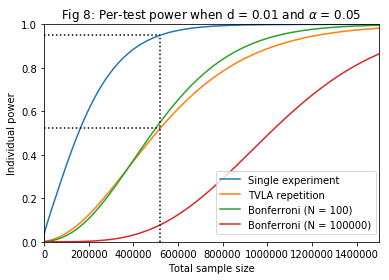We see from the above that the total sample size required to achieve a
95% power in a single experiment only achieves 52% power when the TVLA
repetition step is performed. A Bonferroni correction assuming a trace of
length 100 has a similar impact to the repetition method, while with
100,000 trace points it is considerably less powerful. This is really
problematic for the overall goals of evaluation, especially as it might
well be the case that false negatives are actually more of a concern than
false positives. The question then arises of whether or not there exist
alternative ways of dealing with multiple comparisons that don’t have
such a devastating impact on detection rates. One possibility is to
revisit the independence assumption; aside from being unrealistic, it
also leads to conservative significance criteria which penalise the
detection rates more than is necessary. Better understanding about the
this is outside of scope for this tutorial.

The negative impact of multiple testing is mitigated to some extent if we
are willing to revise down the notion of ‘overall detection rate’ that we
care about. The probability of detecting all true leaks will be even
lower than the power of each individual test; however, the probability of
finding at least one leak grows as the number of true leaks increases,
so that testing more points has the potential to help as well as harm the
detection rate by that definition. Suppose, for example, that there are
20 ‘very small’ leaks in a large dataset:

In :
#Test scenario:
numleaks = 20
alpha = 0.05
numtrace = 2500000
stdes = 0.01
inc = 100

#Compute the power at each sample size increment:
power = np.zeros((numtrace//inc,2))

for n in range(inc, numtrace, inc):
power[n//inc,0] = sp.norm.cdf( (np.sqrt( (n*stdes**2)/4 ) - sp.norm.ppf(1 - alpha/2) ) );
power[n//inc,1] = sp.norm.cdf( (np.sqrt( (0.5*n*stdes**2)/4 ) - sp.norm.ppf(1 - alpha/2) ) )**2;

#Probability of at least 1 is 1 - probability of none:
power_1min = 1 - (1-power)**numleaks
power_all = power**numleaks

#Legend labels:
legtext = []
legtext.append('Individual power')
legtext.append('Detect all 20')
legtext.append('Detect at least 1')

#Make graph:
plt.figure(fignum)
plt.clf()
plt.plot(np.arange(inc, numtrace+inc, inc), power[:,1])
plt.plot(np.arange(inc, numtrace+inc, inc), power_all[:,1])
plt.plot(np.arange(inc, numtrace+inc, inc), power_1min[:,1])
plt.xlim(inc, numtrace+inc)
plt.ylim(0, 1)
plt.legend(legtext, bbox_to_anchor=(0.5,0.35))
plt.xlabel('Total sample size')
plt.ylabel('Power')
plt.title(r'Fig %.0f: TVLA repetition; d = %g and $\alpha$ = %g' % (fignum,stdes,alpha))
sample_s_cert = inc*np.min(np.where(power_1min[:,1]>0.99))
sample_s_map = inc*np.min(np.where(power_all[:,1]>0.99))
print('Sample size to "certify" leakage (i.e. to find at least one leak): %.0f' % sample_s_cert)
print('Sample size to "map" leakage (i.e. to find and locate all the leaks): %.0f' % sample_s_map)

Sample size to "certify" leakage (i.e. to find at least one leak): 271800
Sample size to "map" leakage (i.e. to find and locate all the leaks): 2367000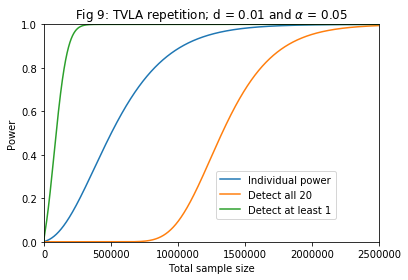## Conclusion

• Ignore false negatives at your peril: naive applications of the TVLA
framework risk prioritising the avoidance of false positives at severe
cost to the testâ€™s ability (‘power’) to detect true leakages.
• Be realistic about the goals of your evaluation: it is possible to
configure a test to be relatively confident of finding at least one leak,
but TVLA cannot be used to ‘find all leaks’.
• Donâ€™t claim more than your test really shows: failure to find a leak
does not prove the absence of leaks. You can never show the strength of a
device against an attack, you can only show (or fail to show) the
weakness of it.

[Coh1988] J. Cohen. Statistical power analysis for the behavioral sciences. Routledge, 1988.

[Saw2009] S. S. Sawilowsky. New effect size rules of thumb. Journal of Modern Applied Statistical Methods, 8(2):597{599, 2009.

[DEM2018] T. De Cnudde, M. Ender, and A. Moradi. Hardware Masking, Revisited. CHES, 2018(2):123-148.

#### Authors:

D. Bellizia {davide.bellizia@uclouvain.be}, UniversitÃ© Catholique de Louvain, ICTEAM/Crypto Group

C. Whitnall {carolyn.whitnall@bristol.ac.uk}, University of Bristol, Dep. of Computer Science/Cryptographic Group

REASSURE (H2020 731591) http://reassure.eu/